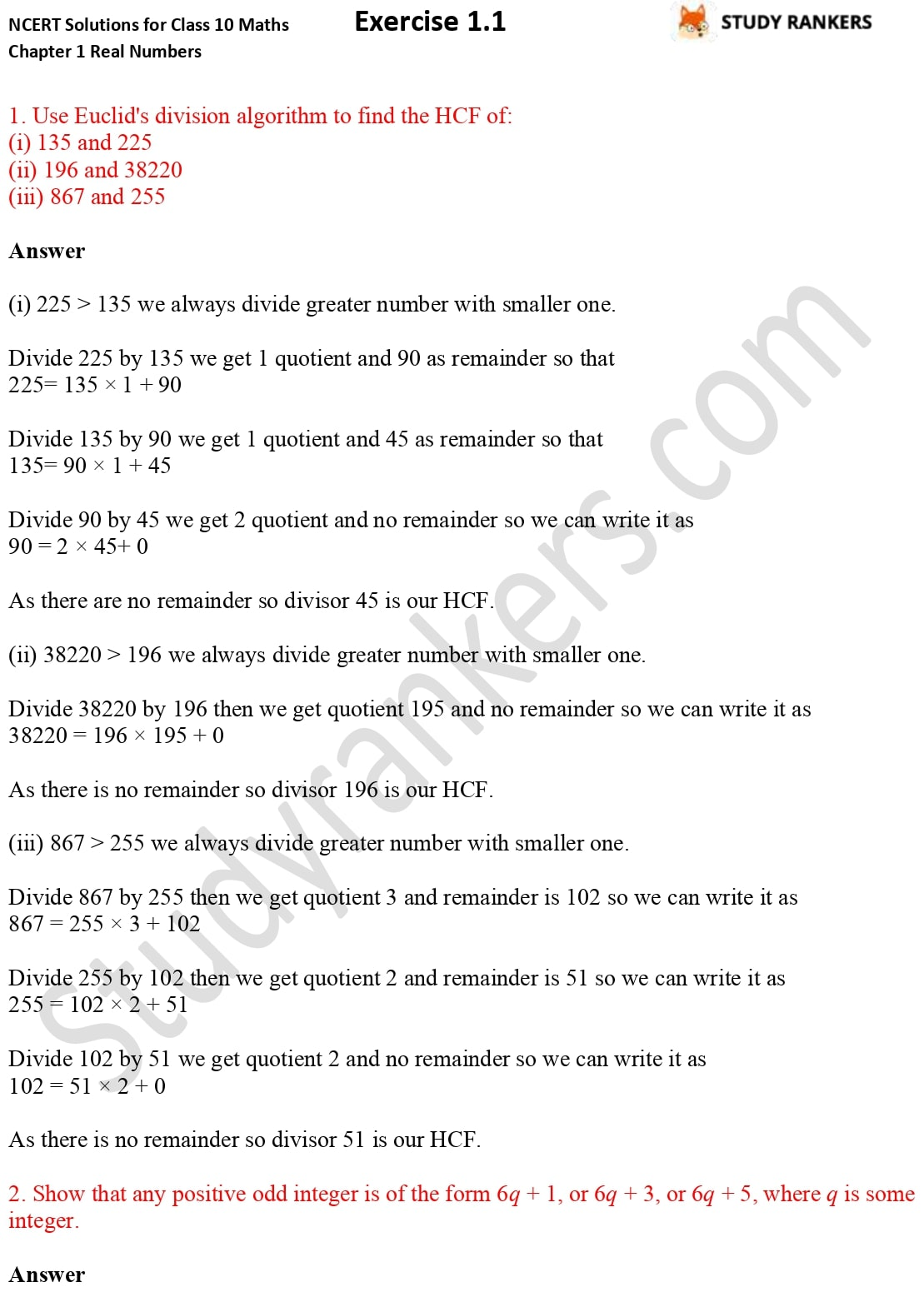## NCERT Solutions for Class 10 Maths Chapter 1 Real Numbers Exercise 1.1

We have provided NCERT Solutions for Class 10 Maths Chapter 1 Real Numbers Exercise 1.1 (Ex 1.1) that will help you solving the exercise and understanding the concepts. These are prepared as per the latest pattern released by CBSE. If you want to view NCERT solutions offline then you can download Studyrankers app from Playstore.

To get all NCERT Solutions for Class 10 Maths follow the link and be updated. In this exercise, we have to find HCF by using Euclid's division algorithm and use Euclid's division lemma to show that the cube of any positive integer is of the form 9m, 9m + 1 or 9m + 8.Chapter 1 Real Numbers Exercise 1.1

The questions in Class 10 Maths Exercise 1.1 Chapter 1 are from Euclid’s Division Lemma which states that in given positive integers a and b, there exist unique integers q and r satisfying a = bq + r, 0  ≤ r < b. A lemma is a proven statement used for proving another statement. Euclid’s division algorithm is based on this lemma. This algorithm is a technique to compute the Highest Common Factor (HCF) of two given positive integers. There are various steps to obtain the HCF of two positive integers, say c and d, with c > d, through this algorithm:
• Step 1 : Apply Euclid’s division lemma, to c and d. So, we find whole numbers, q and r such that c = dq + r, 0 ≤ r < d.
• Step 2 : If r = 0, d is the HCF of c and d. If r ≠ 0, apply the division lemma to d and r.
• Step 3 : Continue the process till the remainder is zero. The divisor at this stage will be the required HCF.
This algorithm works because HCF (c, d) = HCF (d, r) where the symbol HCF (c, d) denotes the HCF of c and d, etc. By following this algorithm, you have to solve Exercise 1.1.

### NCERT Solutions for Chapter 1 Real Numbers Exercise 1.1

1. Use Euclid’s division algorithm to find the HCF of :
(i) 135 and 225 (ii) 196 and 38220 (iii) 867 and 255

2. Show that any positive odd integer is of the form 6q + 1, or 6q + 3, or 6q + 5, where q is
some integer.

3. An army contingent of 616 members is to march behind an army band of 32 members in a parade. The two groups are to march in the same number of columns. What is the maximum number of columns in which they can march?

4. Use Euclid’s division lemma to show that the square of any positive integer is either of the form 3m or 3m + 1 for some integer m.

5. Use Euclid’s division lemma to show that the cube of any positive integer is of the form
9m, 9m + 1 or 9m + 8.

You will find NCERT Solutions for Chapter 1 Exercise 1.1 above which are detailed and accurate. In order to find NCERT Solutions of other exercises, you can follow provided links.

These Maths Class 10 solutions will help you getting more marks in the examination as these are prepared accoring to the latest pattern 2019-20 released by CBSE.